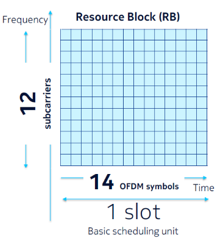# 5G Thrpt Calculation

Interviewer:
When using the 30khz subcarrier spacing ,what is the throughput associated with a resource allocation of 100 resource block across 1 slot when 1 symbol is reserved for the PDCCH and 1 symbol is reserved for the DMRS ?(assuming 256QAM dual stream 2x2 MIMO).

Thrpt= [2 * 6 * (12 * 100) *14]/0.001=201.6Mbps
Thrpt= [2 * 6 * (12 * 100) * 12]/0.001=172.8Mbps
Thrpt= [2 * 8 * (12 * 100) *14]/0.0005=537.6Mbps
Thrpt= [2 * 8 * (12 * 100) * 12]/0.0005=460.8Mbps

Interviewee: !!!
Let’s think together & let’s start from its end.
1- 2*2 MIMO==> so we will have 2 data streams “2”.

2- 256 QAM modulation schemes==> it refers to the number of bits representing one RE (QPSK 2 bits, 16QAM 4 bits, and 64QAM 6 bits, 256QAM 8 bits) so for 256QAM it will be “8bits”.

3- 100 RB with 2 symbols reserved for control information PDCCH & DMRS==> 1 RB means (12 Subcarrier*14 symbols). So, for our question excluding 2 symbols, it will be [100 *12 subcarrier * (14-2) symbols] = “100 * 12 * 12”.

4-1 slot using SCS 30khz==> with SCS =30khz, slot duration is 0.5ms as mentioned on our previous post (#Enjoy_5G_learning__#8). So, to calculate the thpt we have to divide by the time which is 0.5ms =”0.0005s”.